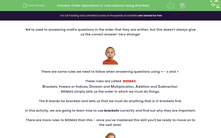# Order Operations in Calculations Using Brackets

In this worksheet, students will begin to learn how to use the rules of BIDMA/BODMAS through the use of brackets.Key stage:  KS 2

Curriculum topic:   Number: Addition, Subtraction, Multiplication and Division

Curriculum subtopic:   Carry Out Calculations Using Order of Operations

Difficulty level:#### Worksheet Overview

We're used to answering maths questions in the order that they are written, but this doesn't always give us the correct answer! Very strange!There are some rules we need to follow when answering questions using + - x and ÷

These rules are called  BIDMAS

Brackets, Powers or Indices, Division and Multiplication, Addition and Subtraction

BIDMAS simply tells us the order in which we must do things.

The B stands for brackets and tells us that we must do anything that is in brackets first.

In this activity, we are going to learn how to use brackets correctly and find out why they are important.

There are more rules to BIDMAS than this - once you've mastered this skill you'll be ready to move on to the next level.Let's have a look at some questions using brackets:

6 + (9 ÷ 3) = 6 + 3 = 9 (brackets first, so do 9 ÷ 3 = 3 first)

7 + (8 x 2) = 7 + 16 = 23 (brackets first, so do 8 x 2 = 16 first)

(12 ÷ 4) + 10 = 3 + 10 = 13 (brackets first, so do 12 ÷ 4 = 3 first)

3 x  (7 - 6) = 3 x 1 = 3 (brackets first, so do 7 - 6 = 1 first)

Let's see how brackets can affect an answer.

Here's a calculation written out twice with exactly the same numbers and symbols, but with the brackets in different places.

Look at how this affects our answer!!

3 x (6 - 3) =  3 x 3 = 9

(3 x 6) - 3 = 18 - 3 = 15

Quite a difference, right?

Let's put (3 x 6) - 3 = 15 into a word problem:

Three children each had 6 sweets:  3 children x 6 sweets = 18 sweetsOn the way to school, they lost 3:

18 sweets - 3 sweets

How many sweets did they have left in total?  = 15

Now, why don't you have a go at answering some similar questions?

Come back and have another look at this page if you get stuck by clicking on the red help button on the screen.

### What is EdPlace?

We're your National Curriculum aligned online education content provider helping each child succeed in English, maths and science from year 1 to GCSE. With an EdPlace account you’ll be able to track and measure progress, helping each child achieve their best. We build confidence and attainment by personalising each child’s learning at a level that suits them.

Get started••••